Tutorials
An explanation as to colour depth and a demonstration of 16 bit advantage over 8 bit

# A bit about Colour Depth and Bits!

04/02/2013
Colour Bit Depth Explained

The sensor in your camera is not digital it is effectively analogue. It receives light (photons) and turns them into electrons. That charge is amplified and then turned into a digital signal using an Analogue to Digital convertor. It is at that point your camera becomes digital.

The number of bits defines the number of levels of that analogue signal (light) that can be measured.

A digital number comprises 0’s and 1’s and the 1‘s like a 2 can change its value depending on where it sits in the number it can be 2 or 20 or 200 or 2000 so can the 1’s but instead of going up in powers of 10 it goes up in powers of 2.

 8 4 2 1 0 0 0 0 = 0 0 0 0 1 = 1 2^0 0 0 1 0 = 2 2^1 0 0 1 1 = 3 0 1 0 0 = 4 2^2 0 1 0 1 = 5 0 1 1 0 = 6 0 1 1 1 = 7 1 0 0 0 = 8 2^3 1 0 0 1 = 9 1 0 1 0 = 10 1 0 1 1 = 11 1 1 0 0 = 12 1 1 0 1 = 13 1 1 1 0 = 14 1 1 1 1 = 15 This is 2^4 - 1

This is a four bit digital number, it can resolve 16 levels, 0 to 15.

So a JPEG image has a maximum of 8 bits per channel. That allows 2^8 or 256 levels per channel.
What is a channel?
Any colour can be represented with a combination of three special colours. In JPEG those have been chosen to be Red (R) Green (G) and Blue (B) (For the purist I know this is not entirely true but it will do for this explanation)
With 3 8 bit channels a total of 16,777,216 colours can be shown.

If you use a 16 bit file format (TIFF, PSD) then the total number of colours that can be represented are 281,474,976,710,656. Note that your camera is likely to be 12 bits (68,719,476,736 colours) or 14 bits (4,398,046,511,104 colours). These levels are internal and they are only available to you if your camera can output a file format other than JPEG.

Note that unless you have a very expensive monitor it will be 3 8 bit channels and unless you have a very expensive printer it will be 3 8 bit channels.

Effect of bits on colour

I will try to show you the effect of bits on colour.
1 Bit (Grey scale)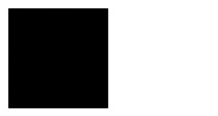0____________1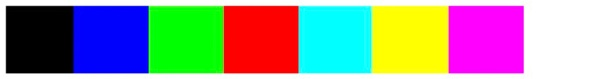R ______ 0 ______ 0 ______ 0 ______ 1 ______ 0 ______ 1 ______ 1 ______ 1 ______

G ______ 0 ______ 0 ______ 1 ______ 0 ______ 1 ______ 1 ______ 0 ______ 1 ______

B ______ 0 ______ 1 ______ 0 ______ 0 ______ 1 ______ 0 ______ 1 ______ 1 ______

I can represent 8 colours which isn’t bad for a 1 bit colour depth!
With 2 bit colour depth we get 64 colours, 3 bit gives 512 colours, 4 bits gives 4096 colours.

Is 16 bit better than 8bit?

Ok. This is what I did.
I created two spaces 400 * 50 pixels (100%) one in 16 bit space and another in 8 bit space.
I then cropped the two spaces at the black end to be 200 * 50 pixels (50%), so the strip went from black to mid grey. I then used levels to stretch the histogram back to the full 0-255.
I repeated this down to 12.5% where the test piece is 50 pixels square.
The differences can be seen in the images below, does this mean 16 bit is better than 8 bit?

I would also add that I’ve never stretched a levels histogram as much as 50% during manipulation!
This experiment shows the difference between 16 bit and 8 bit, remember your camera is probably 12 or 14 bit so the difference will be less.
I am aware of the fact that the image cannot show posterisation as there are insufficient pixels to do so ( 400px 256 levels 100%; 200px 128 levels 50%; 100px 64 levels 25%; 50px 32 levels 12.5%; Expressed as % of original). The histograms during the process showed posterisation.
I did this experiment as I was unable to see a difference between an 8 bit and a 12 bit version of an image where the histogram was stretched from 50% to 100%.
If anyone has an image which shows a difference between the 8 bit version and the full version after manipulation then I’d like to put it here. If anyone believes my experiment has an error please let me know.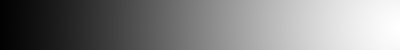100% 16 bit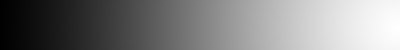100% 8 bit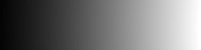50% 16 bit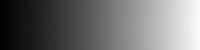50% 8 bit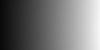25% 16 bit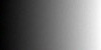25% 8 bit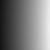12.5% 16 bit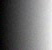12.5% 8 bit